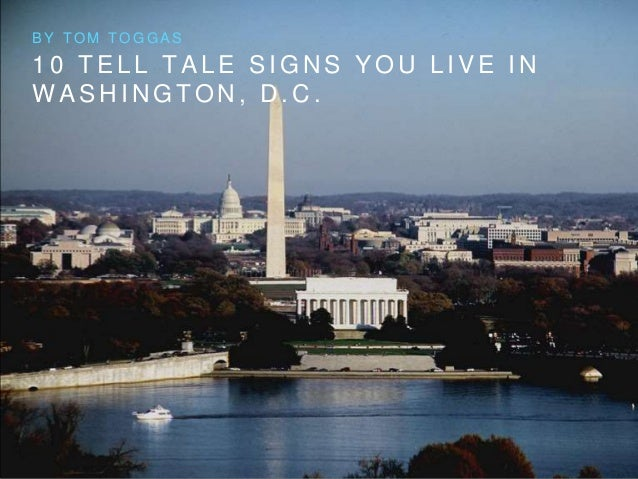Successfully reported this slideshow.Upcoming SlideShare
×

10 Tell Tale Signs You Live in Washington, D.C.

324 views

Published on

If you've spent any regular period of time living and working in Washington, D.C., you can probably relate.

Published in: Entertainment & Humor
• Full Name
Comment goes here.

Are you sure you want to Yes No• Be the first to comment

• Be the first to like this

10 Tell Tale Signs You Live in Washington, D.C.

1. 1. 1 0 T E L L T A L E S I G N S Y O U L I V E I N W A S H I N G T O N , D . C . B Y T O M T O G G A S
2. 2. 1 . W H E N Y O U G O T O A S P O R T S B A R T O W A T C H T H E G A M E , Y O U E N D U P A R G U I N G A B O U T P O L I T I C S .
3. 3. 2 . Y O U K N O W E A S T - W E S T S T R E E T S A R E L E T T E R E D , N O R T H - S O U T H S T R E E T S A R E N U M B E R E D , A N D D I A G O N A L S T R E E T S A R E N A M E D A F T E R S T A T E S .
4. 4. 3 . Y O U D O A L L Y O U R S H O P P I N G O N M S T R E E T .
5. 5. 4 . Y O U ’ V E B E C O M E C O M F O R T A B L E N O T B E I N G T H E S M A R T E S T P E R S O N I N T H E R O O M .
6. 6. 5 . Y O U ’ V E S T O O D I N L I N E F O R G E O R G E T O W N C U P C A K E S , A T L E A S T O N C E .
7. 7. 6 . Y O U ’ R E P R O U D T O S A Y Y O U L O V E T H E D M V . (As long as we’re referring to the acronym used for the greater Washington D.C. area, which includes parts of Maryland and Northern Virginia.)
8. 8. 7 . Y O U ’ V E C A U G H T Y O U R S E L F A S K I N G S O M E O N E W H A T T H E Y D O B E F O R E Y O U ’ V E E V E N A S K E D T H E M T H E I R N A M E .
9. 9. 8 . Y O U ’ V E G O T A T L E A S T 5 P U B L I C F I G U R E S R E A D Y T O N A M E - D R O P A T A N Y G I V E N P O I N T I N A C O N V E R S A T I O N .
10. 10. 9 . T H E R E I S N O W O R S E O F F E N S E T H A N S T A N D I N G O N T H E W R O N G S I D E O F T H E E S C A L A T O R . “Walk left, stand right.”
11. 11. 1 0 . Y O U ’ V E S E E N T H E A I R A N D S P A C E M U S E U M M O R E T I M E S T H A N Y O U C A N C O U N T . ( B E C A U S E E V E R Y O N E W H O V I S I T S Y O U I N S I S T S O N G O I N G , A N D D R A G G I N G Y O U A L O N G . )
12. 12. < 3 W A S H I N G T O N , D . C .